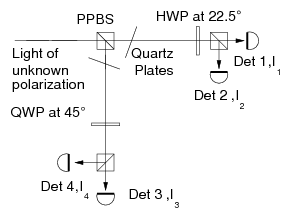# Quantum state tomography

To understand the physical process in a quantum information processing train, and to implement quantum communication schemes without an explicit reference frame it is often necessary to carry out a complete determination of the quantum state of a qubit or a set of qubits.

There have been several implementations of quantum state tomography for photonic qubits and multiqubit states; in this project, we implement a minimal and optimal scheme for this purpose, based on a POVM scheme which recently caught attention both in the quantum information community  and for polarization measurements in classical optics .

The scheme relies on a four-valued measurement outcome for a single photon entering the analyzer, with each of the measurement outcomes corresponding to a polarization or qubit state maximally separated from the states corresponding to the other three detectors; these four states can be represented as a tetrahedron in the Poincare sphere representing the polarisation state of a single photon, or a classical light field:We have three short movies illustrating how such a scheme can be used to determine an unknown polarization state. We use a cylindrical projection of a Poincare sphere, so that we have a "map" of the surface of the sphere. We then prepare three distinct maximally pure polarization states, and accumulate photon numbers at each detector. With each marginal photon, a distribution is built up, and the polarization state can be estimated from this distribution. We accumulate a total of 150 photons. In the movies, the prepared state is marked by a white cross, while the estimated state is marked by a red cross. We have also illustrated a region of arbitrary high likelihood - this region is marked by white. The green line marks the "equator" corresponding to linear polarization states.

We implemented such a measurement scheme using an arrangement of partially polarizing beamsplitters and single photon detectors in a robust experimental setup, allowing us to determine the polarization state both for pure states (or polarized light in classical optics) and mixed states (corresponding to partially polarized light) :With such a scheme, optimal state tomography can be carried out both for single photon states and multiphoton states , allowing to quickly estimate the polarization change e.g. in an optical fiber used for quantum key disribution.

Such a measurement scheme is also useful for an implementatin of a new quantum key distribution protocol, based exactly on correlations between tetrahedral POVMs on both twins of a photon pair in a entangled singlet state .

### References:

  Rehacek, B.-G. Englert, and D. Kaszlikowski, Phys. Rev. A 70, 052321 (2004)  R.M.A. Azzam and F.S. Sudrajat, Appl. Opt. 44, 190-196 (2005)  A. Ling et al., J. Mod. Opt. 53, 1523 (2006)  A. Ling et al., PRA 74, 022309 (2006)  B.-G. Englert, D. Kaszlikowski, H.K. Ng, W.K. Chua, J. Rehacek, and J. Anders, quant-ph/04120752005)